#beanz Magazine

## Bakuro Binary Number Puzzles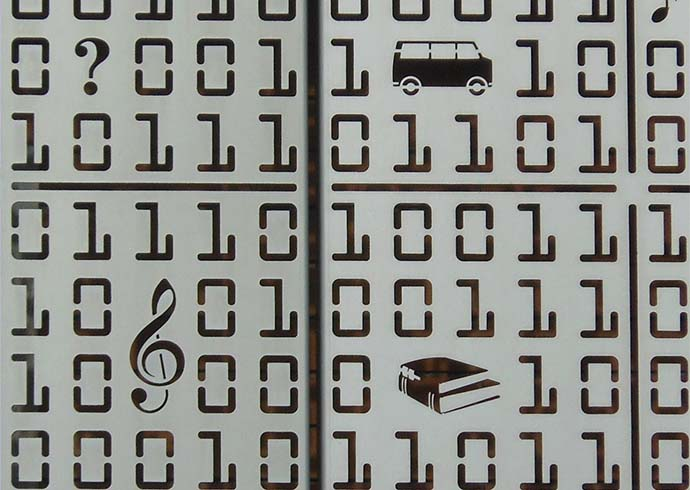mikecogh on Flickr

Bakuro puzzles are a fun way to learn about binary numbers and how to calculate their values.

Bakuro puzzles are like Sudoku puzzles with binary numbers to determine what value to place in empty puzzle squares. It’s a fun way to learn about binary numbers and solve complex puzzles.

Bakuro puzzles are Kakuro puzzles that use binary numbers. Paul Curzon at TeachingLondonComputing.org, with support from Google, has created a set of Bakuro puzzles plus blank forms to create your own. This article describes the basics of Bakuro with links at the bottom to explore more, including Kakuro puzzles.

### What are Binary Numbers?

If you don’t know, in their simplest form, binary numbers use a 1 or 0 to indicate a number value based on their location in a string of binary numbers. Each position in the binary number string — counting from right to left — has a number value of 1, 2, 4, 8, 16, and so on. The position of each 1 in the string is used to add up the value of the binary number.

Here’s an example with a 5-digit binary number:

5      4   3   2   1 <== position of value, counted from right to left
16   8   4   2   1 <== value of each position, as a power of 2

These binary numbers and their values are added up based on the position of the 1 within the 5-digit binary number:

00001 = 1
00011 = 2 + 1 = 3
00111 = 4 + 2 + 1 = 7
01111 = 8 + 4 + 2 + 1 = 15

Do you see how these binary numbers equal one less than the value of the next position in the binary number? 00111 equals 7 while 01000 equals 8. And 00011 equals 3 while 00100 equals 4. Binary numbers are interesting.

To convert numbers into binary numbers, start with the nearest power of 2 represented in the binary number. For example, to convert the number 6 into binary, the nearest power of 2 is 4 and leaves you with a remainder of 2 (6 – 4). The remainder of 2 can be converted into binary with a 1 placed in the second position, counting from the right of the binary number, as shown here:

0      0   1   1   0 = 4 + 2 = 6
16   8   4   2   1 <== value of each position, as a power of 2
5      4   3   2   1 <== position of value, counted from right to left

### How to Solve a Basic Bakuro Binary Numbers Puzzle

With this basic understanding of how binary numbers are calculated and how they work, you can start to solve basic Bakuro puzzles like this one:

In a Bakuro puzzle, empty cells in the grid must be filled only with powers of 2: 1, 2, 4, and 8 in this case with 4-digit binary numbers. The numbers or blocks must equal the number given in the binary number clue at the top and left of the puzzle. In the puzzle above, for example, the first column cells must equal the binary number 1001 which converts to 9. The two 1 digits are in the 8 and 1 position, as explained above.

Here’s how to work this simple Bakuro puzzle to a solution.

Because you’re smart, you’ll realize the value of each position of a 1 digit tells you what numbers to enter in the cell (but not in what order: you have to look at the clue on the left of each row to learn the correct order). In the simple Bakuro example below, your top left most empty column cell must include an 8 or a 1 because the 1 digits are in the 8 and 1 positions. The 1001 binary number adds up to 8 + 1 or 9.

Now look at the far left column and the 0011 and 1100 numbers. The top row binary number converts to 3 (0011) and the bottom row converts to 12 (1100). For 0011, the 1 digits are in the 1 and 2 value positions. For 1100, the 1 digits are in the 4 and 8 value positions.

In the top row, we need to figure out how to divide up the left column 1001 binary number, which converts to 8 and 1. The top left row 0011 binary number tells us the row values are either a 1 or a 2 because the 1 digits are in the columns with value of 1 and 2 (1+2=3). We can put a 1 in the top left empty cell with some confidence.

The bottom row 1100 binary number tells us that row uses an 8 or 4 because the 1 digits are in the binary number columns with a value of 4 and 8 (8+4=12).

Therefore, we can say the top left empty cell should be filled with 1 and the bottom left empty cell should be filled with an 8. This equals 1001 or 9 at the top of the left column.

This leaves us with the right empty column to fill. With a 1 in the top left solution cell and an 8 in the bottom left solution cell, we need the top row to equal 3 and bottom row equal 12.

The 0011 binary number for the top row has the 1 digits in the 2 and 1 value positions. Therefore, the top right empty cell must be a 2. The top left empty cell already has a 1.

The 1100 binary number for the bottom row works the same way. The 1 digits are in the 8 and 4 value positions. The bottom right empty cell, therefore, must be a 4. The bottom left empty cell already has an 8.

We can double check this solution by calculating the value of the right column. The binary number is 0110 with the 1 digits in the 4 and 2 value positions. Our right column solution of 2 and 4 equals 6 so we’ve solved this basic puzzle. Congratulations!

### Beyond Basic Bakuro Puzzles

Bakuro puzzles can be as addictive as Sudoku puzzles. However, you get far more geek points because Sudoku uses mere numbers. Anyone can play with numbers. Bakuro requires you to know binary numbers and how to calculate them.

This simple Bakuro puzzle is from the Teaching London Computing website, a resource for teachers, parents, and students. Here’s another puzzle if you want to print and solve it:

All puzzles from Paul Curson at TeachingLondonComputing.org/bakuro with help from Google.

Below are links to more Bakuro puzzles you can find online. These are from Computing at Schools, an organization in the United Kingdom that supports computer science teachers. I’ve also linked to Kakuro puzzles if you want to relax and work with mere numbers.

### Also In The April 2019 IssueUse SketchUp to create this fascinating mathematical pattern that appears everywhere in nature.Learn about the STEAM star’s amazing journey onto Mythbusters Junior and beyond.What activities are best for teaching STEM to young kids?What’s the best way to choose a classroom lunch? Or the best way to elect a leader? The answer isn’t so simple.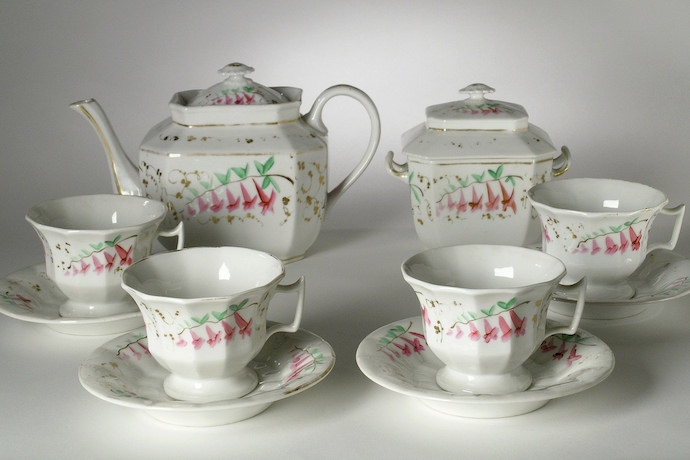How a teapot became the most important object in computer graphics.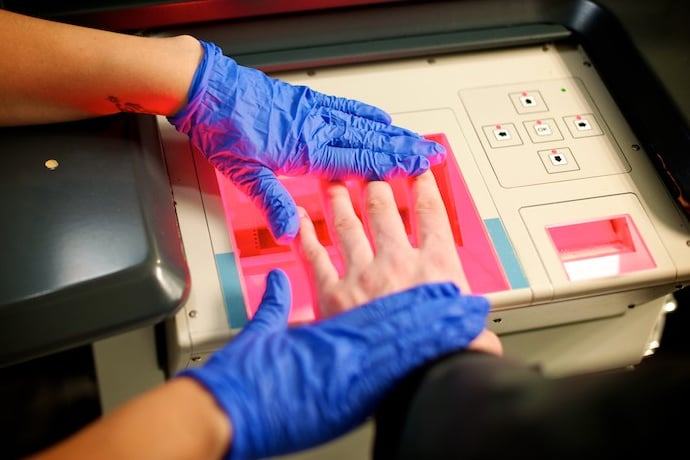Bring your coding skills and your desserts to new levels in this simple Python coding activity.Bakuro puzzles are a fun way to learn about binary numbers and how to calculate their values.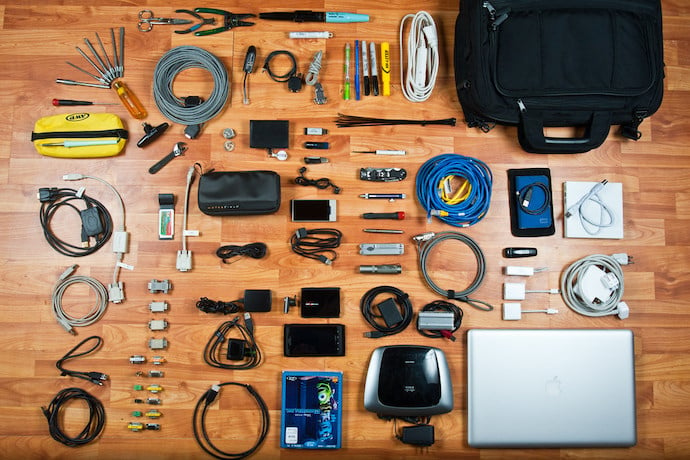Learn about the shiny new technology that allows us to be connected like never before.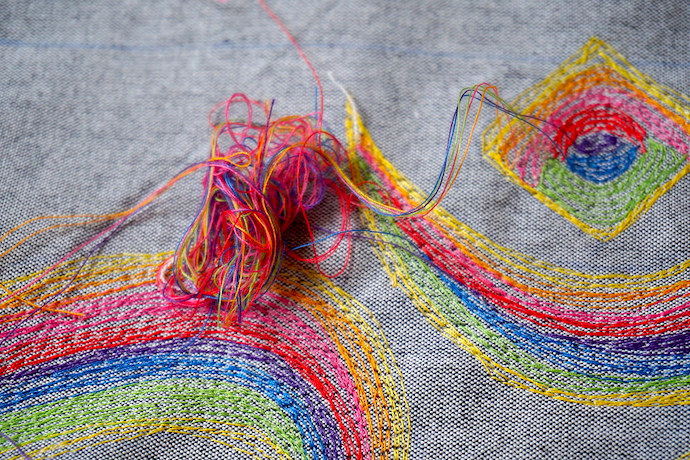Squares, checkerboards, and hollow boxes… what pattens can you imagine in Python?A fun, DIY electronics project that’ll keep you from bumping around in the dark!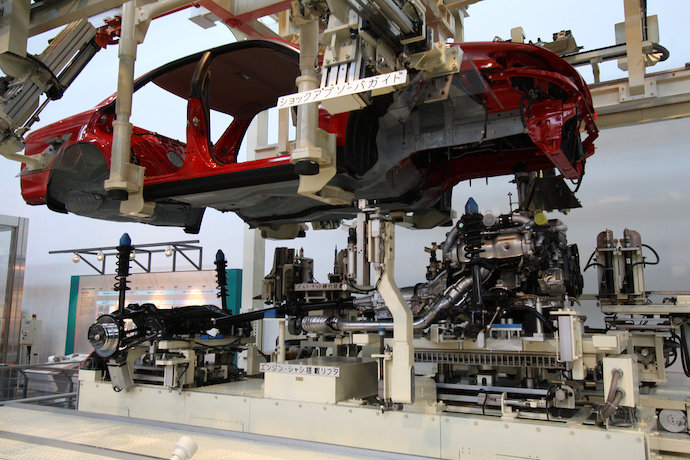It’s time for a throw back to old school programming. Dive into the nuts and bolts of coding instructions!Use your favourite block language to animate this fascinatingly odd game.Can we make a computer using only three simple rules?How science and tech led to an exciting discovery in one of the most dangerous areas of space.How did video games become popular before the internet? It’s all about shareware, floppy disks, and human cleverness!Links from the bottom of all the April 2019 articles, collected in one place for you to print, share, or bookmark.Interesting stories about science and technology for April 2019.